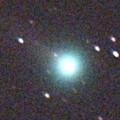# \$B%M%*%o%\$%:WB@1(B

C/2020 F3 ( NEOWISE )###\$B%W%m%U%#!<%k(B

 \$BH/8+F|(B 2020\$BG/(B3\$B7n(B27\$BF|(B \$BH/8+8wEY(B 17\$BEy(B \$BH/8+ Near-Earth Object Wide-field Infrared Survey Explorer

###\$B###\$B50F;MWAG(B

```Epoch 2020 July 10.0 TT = JDT 2459040.5
T 2020 July 3.68133 TT                                  Rudenko
q   0.2947065            (2000.0)            P               Q
z  +0.0027459      Peri.   37.27428     +0.71858402     +0.14393304
+/-0.0000297      Node    61.01114     +0.28198556     -0.95461655
e   0.9991908      Incl.  128.93729     +0.63570524     +0.26074993
From 335 observations 2020 Mar. 27-June 5, mean residual 0".4.
```

###\$B@1?^(B###\$B8wEYJQ2=(B

```        m1 = 3.5 + 5 log\$B&\$(B + 30   log r  [   ,-66]  (              \$B!A(B2020\$BG/(B 4\$B7n(B28\$BF|(B)
m1 = 7.0 + 5 log\$B&\$(B + 12.0 log r  [-66,   ]  (2020\$BG/(B 4\$B7n(B28\$BF|!A(B              )
```##### \$B50F;MWAG\$O(BM.P.E.C. 2020-M114\$B\$K7G:\\$5\$l\$?\$b\$N\$G\$9!#(B \$B@1?^\$O%9%F%i%J%S%2!<%?(B Ver.10 (\$B%"%9%H%m%"!<%D(B) \$B\$G:n@.\$7\$?\$b\$N\$G\$9!#(B \$B8wEY%0%i%U\$O(BComet for Windows\$B\$G:n@.\$7\$?\$b\$N\$G\$9!#(B